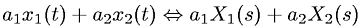Equations > Signal Processing > Laplace Transform Properties > Laplace transform linearity property

### Laplace transform linearity propertyLatex Code:

MathML Code:

 ${a}_{1}{x}_{1}\left(t\right)+{a}_{2}{x}_{2}\left(t\right)⇔{a}_{1}{X}_{1}\left(s\right)+{a}_{2}{X}_{2}\left(s\right)$

MathType 5.0: